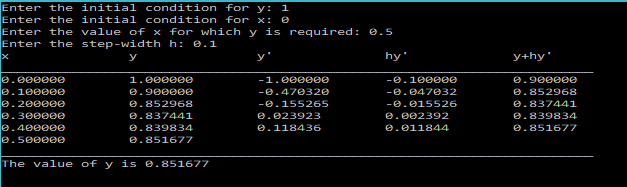Euler Method – C PROGRAM

Euler Method is a Numerical technique used to solve ordinary differential equations.

In this post I will show you how to write a C program to find the solution of a first order differential equation using the Euler’s Method.

Let’s say we have a differential equation$\frac{dy}{dx}=x$
One can easily see that,$y=x^2/2 + C$ is the solution.
Now if we have some initial values for the function, like if we are given$y(0)=1$, then we know that the constant of integration$C=1$.
Using this we can now calculate the value of y for any given x.

This is what we would like to achieve from our program. We cannot have our program tell us the analytical form of y, but we can approximate the value of y for a given x, using the Euler’s Method.

In Euler’s Method, we ask the user to give us the initial values for x and y.
Let’s call these$x_i$ and$y_i$.
Then we find out the slope of$y$ at$x_i$, using the differential equation$\frac{dy}{dx}$.
Then we use this slope to find the value of$y_{I+1}$ at$x_{i+1}=x_i+h$. So basically we approximate the function using a line. Therefore, for better accuracy the value of$h$ should be very small.
We keep on incrementing x and approximating y until we reach the x value for which y is desired.

Algorithm:

1. Enter the initial values of$x$ and$y$(xi and yi respectively).
2. Enter the value of$x$, for which$y$ is to be determined.
3. Enter the width of the interval, ‘$h$’.
4. Do:
y=y0+(h*dy/dx(xi,yi))
yi=y.
xi=xi+h
Until (xi>=x)
5. Print y, which is the solution.

PROGRAM:

/***********************************************
****EULER METHOD FOR DIFFERENTIAL EQUATIONS*****
***********************************************/
#include<stdio.h>
#include<math.h>
/*Define the RHS of the first order differential equation here(Ex: dy/dx=f(x,y))  */
double f(double x, double y){
return -2*x-y;
}
main(){
int i;
double y,xi,yi,xf,h;
printf("Enter the initial condition for y: ");
scanf("%lf",&yi);
printf("Enter the initial condition for x: ");
scanf("%lf",&xi);
printf("Enter the value of x for which y is required: ");
scanf("%lf",&xf);
printf("Enter the step-width h: ");
scanf("%lf",&h);
printf("x\t\ty\t\ty'\t\thy'\t\ty+hy'\n");
printf("__________________________________________________________________________\n");
//Begin Euler Routine
while(xi<xf){
y=yi+h*f(xi,yi);
printf("%lf\t%lf\t%lf\t%lf\t%lf\n",xi,yi,f(xi,yi),h*f(xi,yi),y);
yi=y;
xi=xi+h;
}
printf("%lf\t%lf\n",xi,yi);
printf("__________________________________________________________________________\n");
printf("The value of y is %lf\n\n",y);
}


OUTPUT:$dy/dx=-2x-y$$y'= 2-2y-exp(-4x)$REFERENCES:

http://tutorial.math.lamar.edu/Classes/DE/EulersMethod.aspx